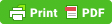# Chapter : Rest and Motion

Simple pendulum(image source: wikipedia)

In our daily life we see many objects that are either in rest position or Motion. eg- moving Trains, car ,cycle, etc and on other hand trees, houses, furnitures, etc are in rest position.

## Rest :-

If any object does not change its position with respect to time and its surroundings, then it is called at rest.

## Motion:-

If the position of any objects changes with the time and its surroundings, then it is said to be at the state of motion.

## Types of motion

### Rectilinear and Translatory motion :-

If a body travels in a straight line, horizontally or vertically, then the motion is called rectilinear or Translatory motion. eg - motion of a car in straight line, falling of a object from some height.

### Circular Motion :-

when a body moves along a circular path, then its motion is called circular motion. In circular motion a body always accelerated towards the gravity.

### Periodic Motion :-

The motion which repeats itself after a regular interval of time is called periodic motion. eg - movement of hands in a clock.

### Simple Harmonic Motion :-

If a body moves to and fro in a straight line about a fixed point or position, then the motion of the body is called Simple Harmonic Motion. eg - movement of a pendulum.

### Oscillatory Motion :-

If a body repeats its movement after a regular interval of time about  a fixed point, then the motion is called Oscillatory motion.

### Projectile Motion :-

Projectile motion is a form of motion in which an object or particle is thrown near the earth’s surface, and its moves along a curved path under the action of gravity. The path of projectile is always parabolic.

For the maximum range of the projectile, the angle of projection should be 45°.

For maximum height of the projection, the angle of projection should be 90°.

## Simple Pendulum :-

A simple Pendulum i s a point mass suspended from rigid support by means of an elastic inextensible string.
Time period of a simple pendulum depends on the length of the string, which is known as effective length, and the acceleration due to gravity.
t = 2 π√l/g
Where l = length of the string and g = acceleration due to gravity.
Time period of a simple pendulum is independent of the mass of the bob which is suspended.

## Some Basic Terms related with Motion

### Distance :-

The length of the path covered by a body in a particular time interval is called diatanced.
Distance is always positive and its unit is meter (m). It is a scalar quantity.

### Displacement :-

The shortest distance between the initial and final points in a particular direction is called displacement.
It can be negative, zero or positive. Its unit is also meter (m). It is a vector quantity.

### Speed :-

The rate of change of distance is called speed.
Mathematically, speed = distance/time.
It is a scalar quantity and its unit is m/s.

### Velocity :-

The rate of change of displacement is called velocity, i.e. Velocity = displacement/time.
It is a vector quantity and its unit is also m/s.It may be negative, zero or positive.

### Uniform velocity :-

If a body covers equal displacements in equal interval of time, then it is said that the body is moving with uniform velocity.

### Non-uniform Velocity :-

If a body covers unequal displacement in equal intervals of time, then it is said that the body is moving with non-uniform velocity.

### Acceleration :-

It is the rate of change of velocity.It is a vector quantity. Its S.I. unit is m/s.
It may be negative, zero or positive.
If it is positive, then it is known as acceleration. If it is zero, then the body is moving with a uniform velocity. If it is negative, then it is known as retardation.

## Equations of Motion

v=u+at
s=ut+1/2at²
v²=u²+2as
Where, s = distance or displacement covered by the body
v = final velocity
u = initial velocity
t = time period
a = acceleration